Next: 5.3 Experiments Up: 5.2 Pattern association algorithm Previous: 5.2.2.1 Examples

## 5.2.3 Recall

In recall, the components of a pattern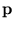are partially given, and the components of a completed pattern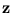are divided into input and output (see section 4.2). The input is the offsetof a hyper-plane extending into the output dimensions: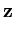=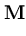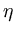+, (5.10)

where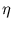are the free parameters of the hyper-plane. The matrix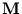defines which dimensions are input and which are output. To obtain the output for a given input, the free parameters are chosen such that the potential E() is minimized,* = arg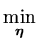E(()) ,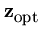=* +. (5.11)

There seems to be no analytical solution for*. In feature space, the constraint space is not a hyper-plane. However, the minimization can be solved using standard numerical optimization algorithms.Next: 5.3 Experiments Up: 5.2 Pattern association algorithm Previous: 5.2.2.1 Examples
Heiko Hoffmann
2005-03-22